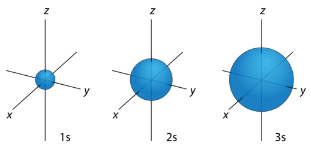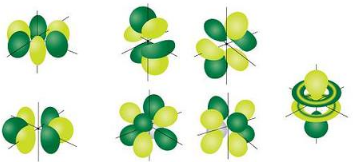# 3.5: Orbital shapes

$$\newcommand{\vecs}{\overset { \rightharpoonup} {\mathbf{#1}} }$$ $$\newcommand{\vecd}{\overset{-\!-\!\rightharpoonup}{\vphantom{a}\smash {#1}}}$$$$\newcommand{\id}{\mathrm{id}}$$ $$\newcommand{\Span}{\mathrm{span}}$$ $$\newcommand{\kernel}{\mathrm{null}\,}$$ $$\newcommand{\range}{\mathrm{range}\,}$$ $$\newcommand{\RealPart}{\mathrm{Re}}$$ $$\newcommand{\ImaginaryPart}{\mathrm{Im}}$$ $$\newcommand{\Argument}{\mathrm{Arg}}$$ $$\newcommand{\norm}{\| #1 \|}$$ $$\newcommand{\inner}{\langle #1, #2 \rangle}$$ $$\newcommand{\Span}{\mathrm{span}}$$ $$\newcommand{\id}{\mathrm{id}}$$ $$\newcommand{\Span}{\mathrm{span}}$$ $$\newcommand{\kernel}{\mathrm{null}\,}$$ $$\newcommand{\range}{\mathrm{range}\,}$$ $$\newcommand{\RealPart}{\mathrm{Re}}$$ $$\newcommand{\ImaginaryPart}{\mathrm{Im}}$$ $$\newcommand{\Argument}{\mathrm{Arg}}$$ $$\newcommand{\norm}{\| #1 \|}$$ $$\newcommand{\inner}{\langle #1, #2 \rangle}$$ $$\newcommand{\Span}{\mathrm{span}}$$$$\newcommand{\AA}{\unicode[.8,0]{x212B}}$$

The flight path of a commercial airliner is carefully regulated by the Federal Aviation Administration. Each airplane must maintain a distance of five miles from another plane flying at the same altitude, and be 2,000 feet above and below another aircraft (1,000 feet if the altitude is less than 29,000 feet). So, each aircraft only has certain positions it is allowed to maintain while it flies. Quantum mechanics demonstrates that electrons have similar restrictions on their locations.

Knowledge of quantum numbers can be applied to describe the arrangement of electrons for a given atom. This application is done by using electron configurations. Electron configurations are effectively a map of the electrons for a given atom. We look at the four quantum numbers for a given electron, and then assign that electron to a specific orbital in the next Module.

## $$s$$ Orbitals

For any value of $$n$$, a value of $$l=0$$ places that electron in an $$s$$ orbital. This orbital is spherical in shape:Figure $$\PageIndex{1}$$: $$s$$ orbitals have no orientational preference and resemble spheres.

## $$p$$ Orbitals

From the table below, it is evident that there are three possible orbitals when $$l=1$$. These are designated as $$p$$ orbitals and have dumbbell shapes. Each of the $$p$$ orbitals has a different orientation in three-dimensional space.Figure $$\PageIndex{2}$$: $$p$$ orbitals have an orientational preference and resemble dumbbells.

## $$d$$ Orbitals

When $$l=2$$, $$m_l$$ values can be $$-2, \: -1, \: 0, \: +1, \: +2$$ for a total of five $$d$$ orbitals. Note that all five of the orbitals have specific three-dimensional orientations.Figure $$\PageIndex{3}$$: $$d$$ orbitals have an orientational preference and exhibit complex structures.

## $$f$$ Orbitals

The most complex set of orbitals are the $$f$$ orbitals. When $$l=3$$, $$m_l$$ values can be $$-3, \: -2, \: -1, \: 0, \: +1, \: +2, \: +3$$ for a total of seven different orbital shapes. Again, note the specific orientations of the different $$f$$ orbitals.Figure $$\PageIndex{4}$$: $$d$$ orbitals have an orientational preference and exhibit quite complex structures.

Orbitals that have the same value of the principal quantum number form a shell. Orbitals within a shell are divided into subshells that have the same value of the angular quantum number. Some of the allowed combinations of quantum numbers are compared in Table$$\PageIndex{1}$$.

Table $$\PageIndex{1}$$: Electron Arrangement Within Energy Levels
Principal Quantum Number $$\left( n \right)$$ Allowable Sublevels Number of Orbitals per Sublevel Number of Orbitals per Principal Energy Level Number of Electrons per Sublevel Number of Electrons per Principal Energy Level
1 $$s$$ 1 1 2 2
2 $$s$$ 1 4 2 8
$$p$$ 3 6
3 $$s$$ 1 9 2 18
$$p$$ 3 6
$$d$$ 5 10
4 $$s$$ 1 16 2 32
$$p$$ 3 6
$$d$$ 5 10
$$f$$ 7 14

## Summary

There are four different classes of electron orbitals. These orbitals are determined by the value of the angular momentum quantum number $$l$$.